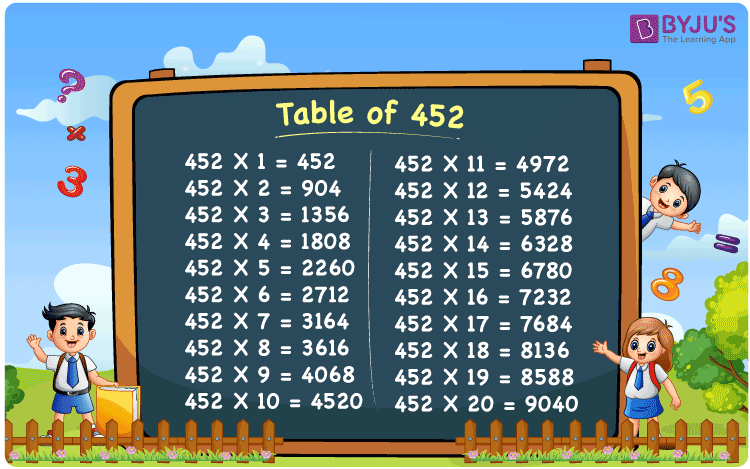Checkout JEE MAINS 2022 Question Paper Analysis : Checkout JEE MAINS 2022 Question Paper Analysis :

# Table of 452

The table of 452 is given here to assist students in checking the table of 452 values up to 20 times. The 452 times table is shown in the tabular format in this article. Download the PDF to get the multiplication table of 452, which is also available on our page. The table of 452 chart will be used as a flashcard to assist students learn and remember the values quickly.

## Table of 452 Chart## What is the 452 Times Table?

Below is the multiplication and repeated addition table of 452:

 452×1 = 452 452 452×2 = 904 452 + 452 = 904 452×3 = 1356 452 + 452 + 452 = 1356 452×4 = 1808 452 + 452 + 452 + 452 = 1808 452×5 = 2260 452 + 452 + 452 + 452 + 452 = 2260 452×6 = 2712 452 + 452 + 452 + 452 + 452 + 452 = 2712 452×7 = 3164 452 + 452 + 452 + 452 + 452 + 452 + 452 = 3164 452×8 = 3616 452 + 452 + 452 + 452 + 452 + 452 + 452 + 452 = 3616 452×9 = 4068 452 + 452 + 452 + 452 + 452 + 452 + 452 + 452 + 452 = 4068 452×10 = 4520 452 + 452 + 452 + 452 + 452 + 452 + 452 + 452 + 452 + 452 = 4520

## Multiplication Table of 452

The table of 452 up to 20 times is given below:

 452 × 1 = 452 452 × 2 = 904 452 × 3 = 1356 452 × 4 = 1808 452 × 5 = 2260 452 × 6 = 2712 452 × 7 = 3164 452 × 8 = 3616 452 × 9 = 4068 452 × 10 = 4520 452 × 11 = 4972 452 × 12 = 5424 452 × 13 = 5876 452 × 14 = 6328 452 × 15 = 6780 452 × 16 = 7232 452 × 17 = 7684 452 × 18 = 8136 452 × 19 = 8588 452 × 20 = 9040

## Solved Example on the Table of 452

Example:

If the cost of one shirt is Rs. 452, what will be the cost of 6 such shirts?

Solution:

The cost of 1 shirt = Rs. 452.

Thus, the cost of 6 such shirts = 6 × 452 = 2712.

Therefore, the cost of 6 such shirts = Rs. 2712.

## Frequently Asked Questions on the Table of 452

### What is the table of 452?

The table of 452 gives the multiples of 452. The table of 452 is also known as the 452 times table.

### Write the table of 452 up to 10 times.

452×1 = 452, 452×2 = 904, 452×3 = 1356, 452×4 = 1808, 452×5 = 2260, 452×6 = 2712, 452×7 = 3164, 452×8 = 3616, 452×9 = 4068, 452×10 = 4520.

### What is 452 times 12?

452 times 12 is 5424. I.e., 452 × 12 = 5424.